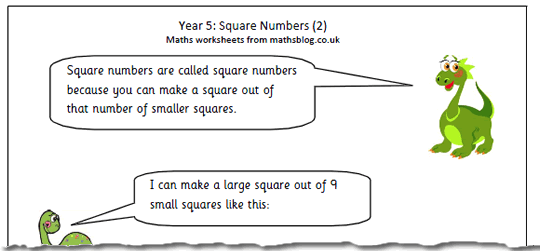# Year 5 Maths Worksheet: Square Numbers (2)This is the second in our series of square number maths worksheets for Year 5.  It is an interesting and worthwhile exercise asking children to see if they can make a square out of 10 or 12 smaller squares (not overlapping etc) using plastic or card squares. Rectangles are possible, but not squares.

They can then be asked to find which numbers can be made into a square. This can be done either with smaller squares or as dots in an array.

There are several ways that questions involving square numbers can be phrased, including:

What is 4 squared?

What is the square of 4?

What number multiplied by itself makes 16?

Square numbers 2